# SAT Math : How to order fractions from least to greatest or from greatest to least

## Example Questions

2 Next →

### Example Question #11 : How To Order Fractions From Least To Greatest Or From Greatest To Least

Order the following fractions from least to greatest: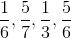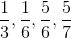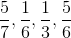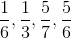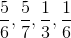Explanation:

To solve this problem, let's find the least common denominator for our fractions.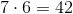is the lowest number that each of our current denominators are factors of.

Now, let's rewrite each fraction so that its denominator is.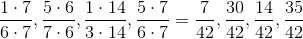Then we can rearrange these new fractions from least to greatest, keeping in mind what they're reduced forms were.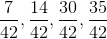Then, by either simplifying these fractions or by remembering their original forms, we can see that the order of our fractions from least to greatest is:### Example Question #12 : How To Order Fractions From Least To Greatest Or From Greatest To Least

Which of the following fractions is the smallest?

1/3, 115/276, 112/350, 1050/3330, 0.75/2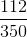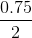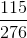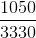Explanation:

1/3, 115/276, 112/350, 1050/3330, 0.75/2

First you need to put the fractions in ascending order:

1050/3330, 112/350, 1/3, 0.75/2, 115/276

Then choose the fraction with the smallest value (1050/3330).

### Example Question #13 : How To Order Fractions From Least To Greatest Or From Greatest To Least

Order from greatest to least: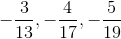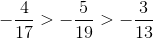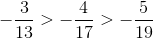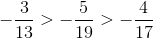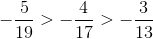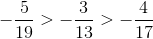Explanation:

Rewrite the numbersin terms of the least common denominator, which is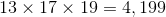: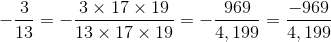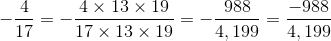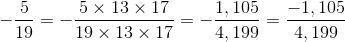Comparing numerators, we see that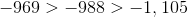It follows that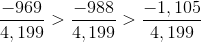and,

making this the correct order.

### Example Question #11 : How To Order Fractions From Least To Greatest Or From Greatest To Least

Order from least to greatest: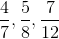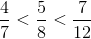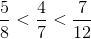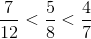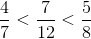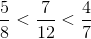Explanation:

Express all three fractions in terms of their least common denominator - the least common multiple of denominators 7, 8, and 12, which is 168. Do this by multiplying the numerator and denominator in each fraction by whatever number yields a product of 168 in the denominator.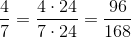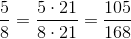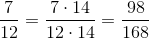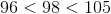it follows that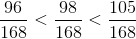,

so### Example Question #15 : How To Order Fractions From Least To Greatest Or From Greatest To Least

Which of the following five numbers is the greatest?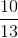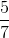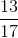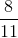Explanation:

For each fraction, divide the numerator by the denominator. This is shown below for each fraction to three decimal places: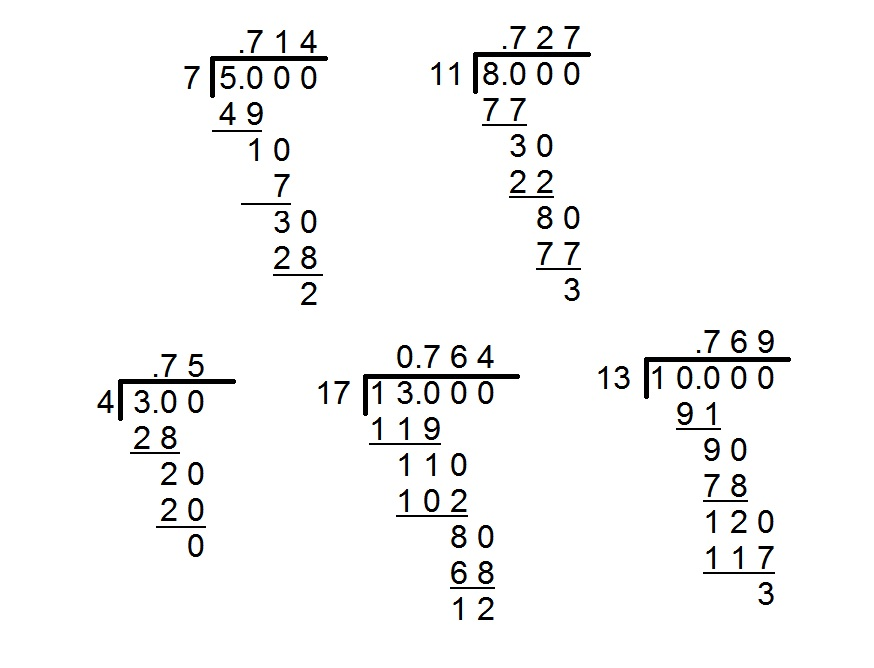From the decimal representations,can be seen to be the greatest of the five choices.

2 Next →

### All SAT Math Resources